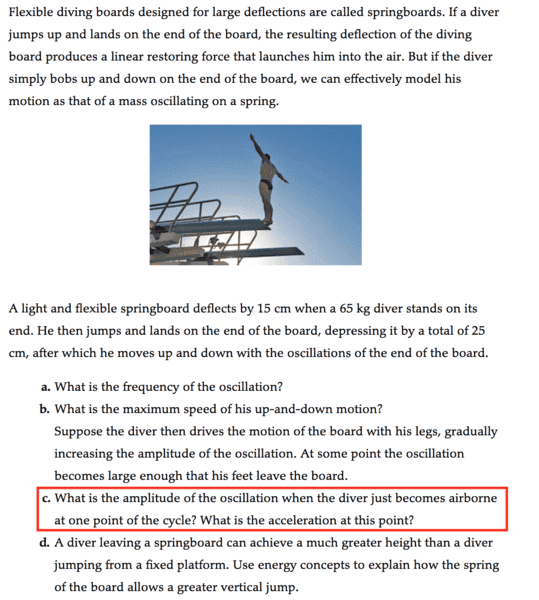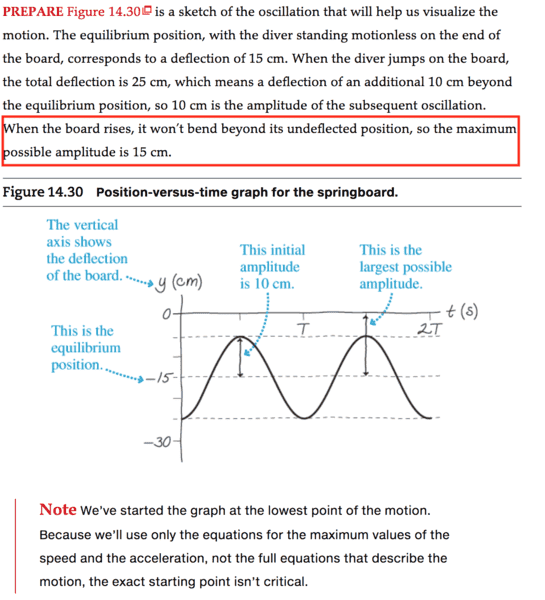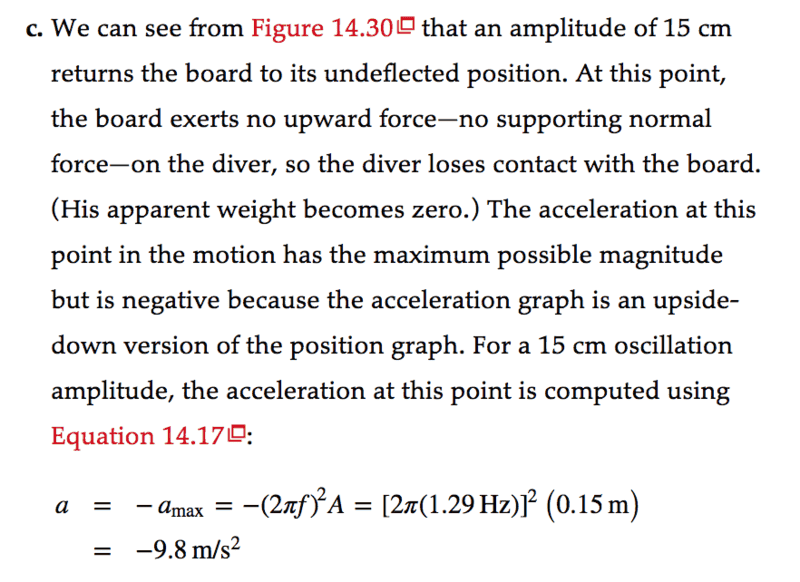# Driven Oscillation: Springboard diving

• Beth N
In summary, the amplitude of the oscillation when the diver just become airborne is v = kA and the acceleration is sqrt (k m ).f

## Homework Statement

A light springboard deflects 15cm when a 65kg diver stands on its end. He jumps up and down, depressing it by 25 cm, then he moves up and down with the oscillations of the end of the board.
What is the amplitude of the oscillation when the diver just become airborne? What is the acceleration at this point?
(in solving problem c, assuming that we have acquired the frequency and the maximum speed from question a and b)

Below is the problem. I highlighted in red the question I have. Feel free to skip uneccessary information.## Homework Equations

##a=A * ( 2 \pi f)^2##

##v=\frac 1 2kA^2##

##f=\frac 1 2\pi (\sqrt {\frac k m})##
a is acceleration, A is amplitude, f is frequency, k is spring constant m is mass

## The Attempt at a Solution

[/B]
The big question I have isn't exactly the question c in the problem, but: why we can be sure that the board won't bend beyond its undeflected position? My thought is that maybe there is no energy input, no force pointing upward; the only driving force here is the man's weight. So when the man no longer exert his weight force upon the board, there is no more restoring force by the board on the man opposing his weight. Hence the board return to its equilibrium position at 0 (in picture).

However, am I correct to say that the man is jumping up and down in resonance with the oscillation of the board? Could resonance possibly increase the amplitude beyond point 0? How can I prove this mathematically? (I tried using the equations I listed above but didn't succeed.

#### Attachments

However, am I correct to say that the man is jumping up and down in resonance with the oscillation of the board?
The problem states that
... he moves up and down with the oscillations of the end of the board.
This, to me, means that he is just at the threshold of losing contact at 5 cm below the position "zero" of the plank. Figure 14.30 shows the position of his feet and the plank at any time t. If he is launched in the air, yes the board will go above the zero point, but this is not what is described in the problem.